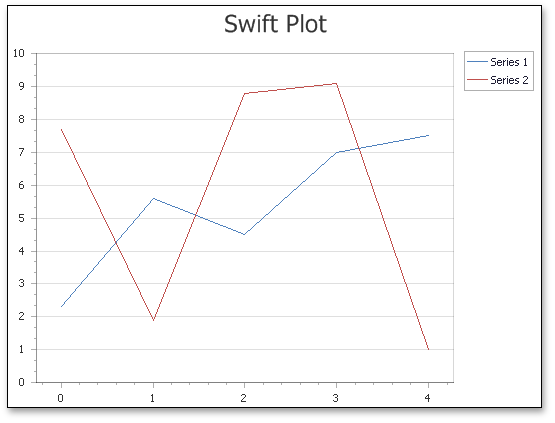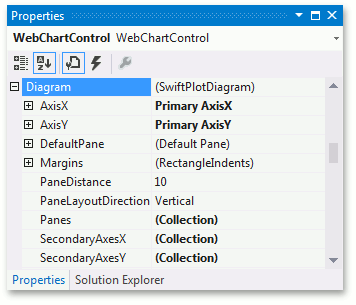20.2
20.1
19.2
19.1
18.2
18.1
17.2
A newer version of this page is available. Switch to the current version.

# Swift Plot Diagram

• 3 minutes to read

This document details the specifics of the Swift Plot diagram type. It describes its purpose and demonstrates how diagram options can be accessed (both at design and runtime). Before reading this text, you may wish to review the basics of using a diagram in the ASP.NET Chart Control.

This document consists of the following sections.

## Swift Plot Overview

The SwiftPlotDiagram object is intended to plot series of only the SwiftPlotSeriesView type. The main purpose of this diagram type is to construct real-time charts in which points are added in a short period of time, and/or charts with a large number of points (tens of thousands and more). To meet these requirements, this diagram type is built upon a special optimized algorithm, with its core being lightened as much as possible, to achieve the best performance.Note that both this diagram and the corresponding series view type still support most features available for other 2D chart types: such as multiple panes, secondary axes, financial indicators and regression lines. So, in most aspects, this diagram type is similar to the XY-Diagram type.

To learn more about Swift Plot charts, refer to Swift Plot.

## Accessing a Diagram

To access the Swift Plot Diagram's options at design time, click your chart, to select it. Then, in the Properties window, expand the WebChartControl.Diagram property.This example demonstrates how to use the SwiftPlotDiagram to display a large data source without freezes.

``````public partial class _Default : Page {
protected void Page_Load(object sender, EventArgs e) {
Series swiftPlotSeries = new Series {
Name = "Swift Plot Series",
View = new SwiftPlotSeriesView()
};
PopulateSeriesWithData(swiftPlotSeries, 100000);
chartControl.Series.Add(swiftPlotSeries);

SwiftPlotDiagram diagram = chartControl.Diagram as SwiftPlotDiagram;
CustomizeSwiftPlotDiagram(diagram);
}

protected void CustomizeSwiftPlotDiagram(SwiftPlotDiagram diagram) {
if (diagram == null) return;
diagram.AxisX.Title.Visibility = DefaultBoolean.True;
diagram.AxisX.Title.Text = "Point Number";
diagram.AxisX.NumericScaleOptions.ScaleMode = ScaleMode.Manual;
diagram.AxisX.NumericScaleOptions.MeasureUnit = NumericMeasureUnit.Custom;
diagram.AxisX.NumericScaleOptions.CustomMeasureUnit = 1000;

diagram.AxisX.NumericScaleOptions.AggregateFunction = AggregateFunction.Average;

diagram.AxisY.Title.Visibility = DefaultBoolean.True;
diagram.AxisY.Title.Text = "Point Value";
}

protected void PopulateSeriesWithData(Series series, int dataPoints) {
Random rnd = new Random();
for (int i = 0; i < dataPoints; ++i) {
series.Points.Add(
new SeriesPoint(
argument: i,
values: 2 * Math.Cos((double)i / dataPoints * 2 * Math.PI)
+ Math.Sin((double)i / dataPoints * 2 * Math.PI)
+ 4 * Math.Cos(2 * (double)i / dataPoints * 2 * Math.PI)
+ 5 * Math.Sin(2 * (double)i / dataPoints * 2 * Math.PI)
+ 0.6 * rnd.NextDouble() - 0.3
)
);
}
}
}
``````